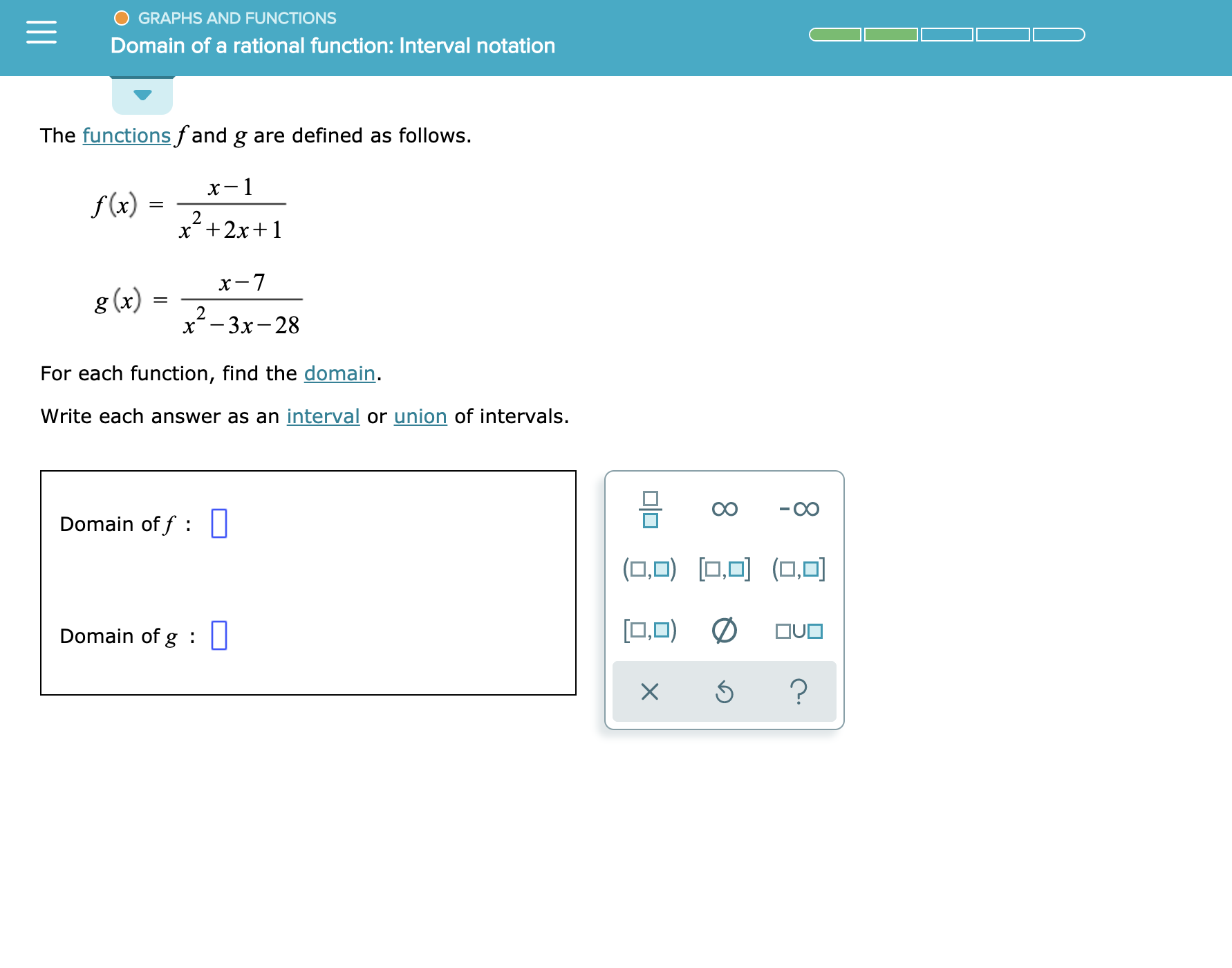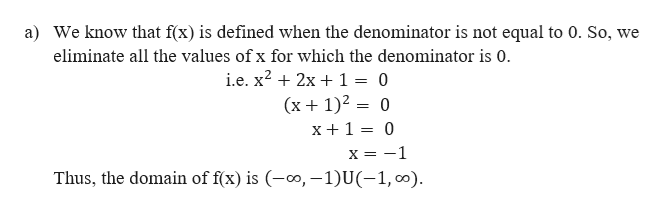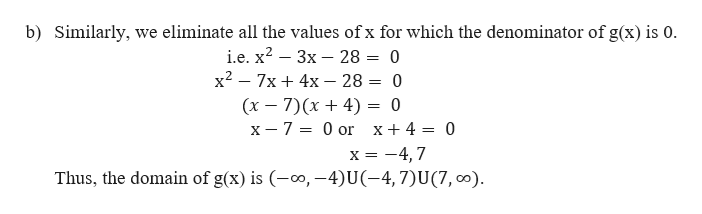# GRAPHS AND FUNCTIONSDomain of a rational function: Interval notationThe functions fand g are defined as follows.х-1f(x)2x2x1х-7g (x)2х* - Зх-28For each function, find the domain.Write each answer as an interval or union of intervals.- CODomain of f||(O (00.O)OUODomain of g :X

Question
11 views

See attachmenthelp_outlineImage TranscriptioncloseGRAPHS AND FUNCTIONS Domain of a rational function: Interval notation The functions fand g are defined as follows. х-1 f(x) 2 x2x1 х-7 g (x) 2 х* - Зх-28 For each function, find the domain. Write each answer as an interval or union of intervals. - CO Domain of f || (O (0 0.O) OUO Domain of g : X fullscreen
check_circle

Step 1

Given functions f(x) ang (x) defined as

Step 2

To find: Domain of the functions.help_outlineImage Transcriptionclosea) We know that f(x) is defined when the denominator is not equal to 0. So, we eliminate all the values of x for which the denominator is 0. i.e. x2 2x 1 = 0 (x 1)20 x+1 0 1 X Thus, the domain of f(x) is -o, -1)U-1, o fullscreen
Step 3

Next,

...help_outlineImage Transcriptioncloseb) Similarly, we eliminate all the values of x for which the denominator of g(x) is o. i.e. x2 3x 28 = 0 x27x 4x - 28 (x 7)(x4) = 0 x- 7 = 0 or 0 x+ 4 = 0 x = -4,7 Thus, the domain of g(x) is o, -4)U(-4, 7)U (7, o) fullscreen

### Want to see the full answer?

See Solution

#### Want to see this answer and more?

Solutions are written by subject experts who are available 24/7. Questions are typically answered within 1 hour.*

See Solution
*Response times may vary by subject and question.
Tagged in
MathCalculus

### Functions